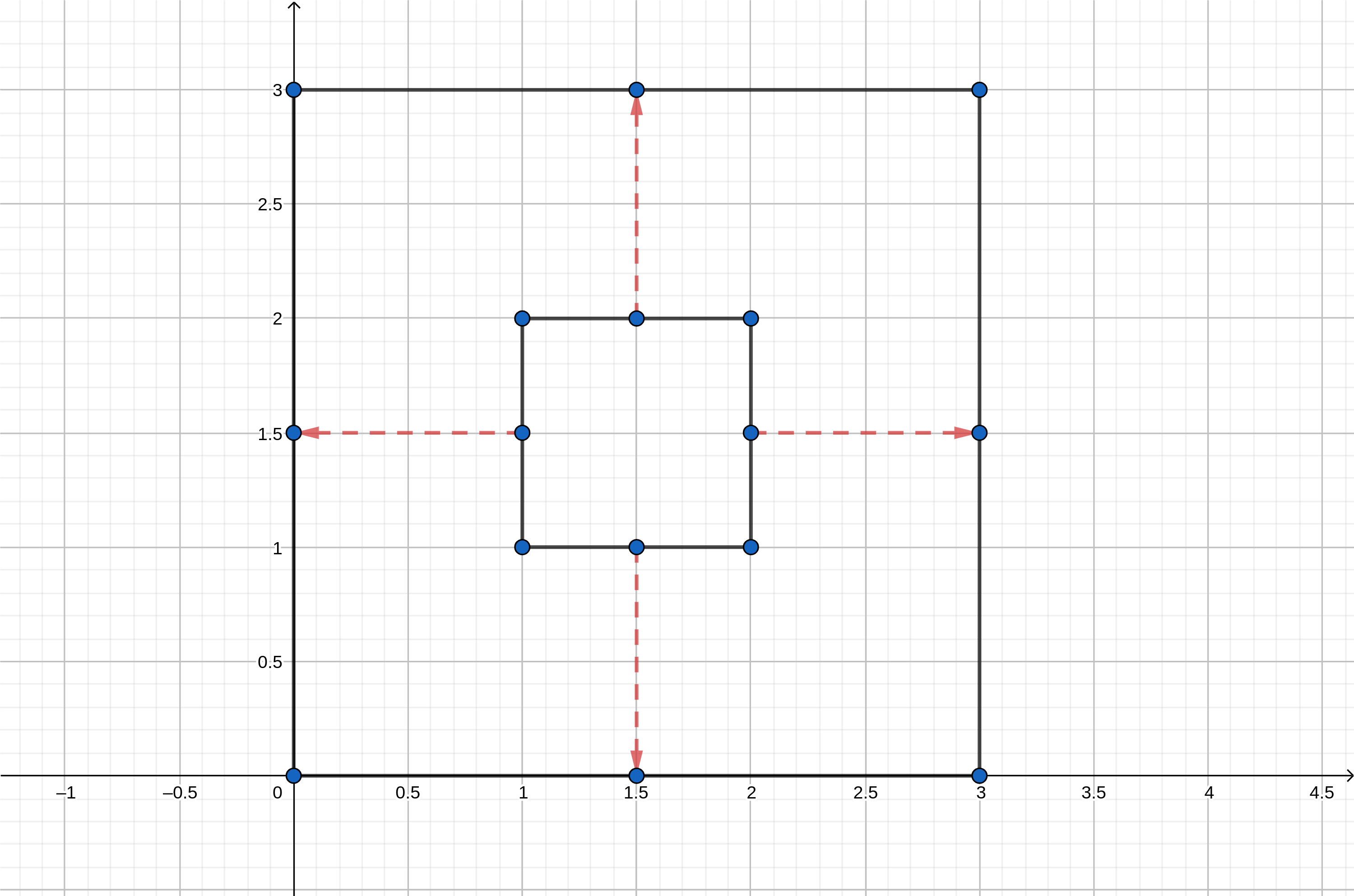Geometry 1 solution codechef

Geometry 1 solution codechef

You are given a convex polygon with NN sides. You have to answer QQ queries. The ithith query is described by two integers vi,tivi,ti. In this query, all sides of the polygon start moving parallel to their respective perpendicular vector away from the centroid with a constant velocity of viunitssecviunitssec. Output the final area covered by NN sides of the polygon after time titi seconds.

For each query, consider the initial coordinates of vertices of the given polygon.

Note:

• Since the input-output is large, prefer using fast input-output methods.
• The problem requires high precision, so prefer using data types with a precision equivalent to long double in C++.

Input Format

• The first line of the input contains a single integer TT denoting the number of test cases. The description of TT test cases follows.
• Each testcase contains N+Q+1N+Q+1 lines of input.
• The first line of each test case contains two space-separated integers N,QN,Q.
• NN lines follow. For each valid ii, the ithith of these lines contains two space-separated integers xi,yixi,yi, coordinates of the ithith vertex of the polygon.
• Next QQ lines follow. For each valid ii, the ithith of these lines contains two space-separated integers vi,tivi,ti, description of the ithith query.

Output Format

For each query, output in a single line the final area covered by NN sides of the polygon.

Your answer will be considered correct if its absolute error does not exceed 10210−2.

Constraints

• 1T1001≤T≤100
• 3N1043≤N≤104
• 1Q1041≤Q≤104
• 0xi,yi21060≤xi,yi≤2⋅106
• 1vi,ti1051≤vi,ti≤105
• 1viti1051≤vi⋅ti≤105
• The sum NN over all testcases does not exceed 51055⋅105.
• The sum QQ over all testcases does not exceed 51055⋅105.
• The vertices of polygon are given in counter-clockwise order.
• The internal angles of the given polygon are greater than 11∘.

Subtask #1 (100 points): original constraints

2
4 1
1 1
2 1
2 2
1 2
1 1
3 2
1 1
2 1
1 2
1 1
2 3

9.0000000
9.7426406
230.8086578

Explanation

Below are the images for the respective test cases. Inner polygons are the ones at the start and the rays represent the distance traveled by the sides at the given speed in a given time. Outer polygons denote the final ones after the movements of the sides.

Test case 11:Test case 221st1st query